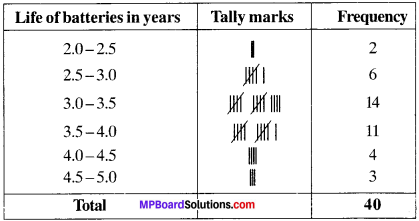## MP Board Class 9th Maths Solutions Chapter 14 Statistics Ex 14.2

Question 1.
The blood groups of 30 students of Class VIII are recorded as follows:

A, B, O, O, AB, O, A, O, B, A, O, B, A, O, O,
A, AB, O, A, A, O, O, AB, B, A, O, B, A, B, O.

Represent this data in the form of a frequency distribution table. Which is the most common, and which is the rarest, blood group among these students?
Solution:
Frequency distribution table: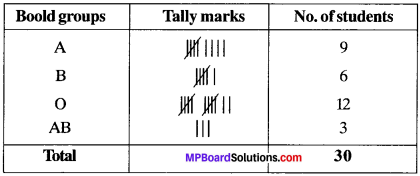Common blood group – O
Rarest blood group – AB.Question 2.
The distance (in km) of 40 engineers from their residence to their place of work were found as follows: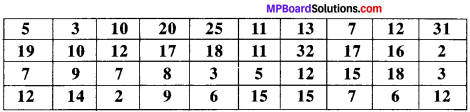Construct a grouped frequency distribution table with class size 5 for the data given above taking the first interval as 0 – 5 (5 not included). What main features do you observe from this tabular representation?
Solution:
Frequency distribution table: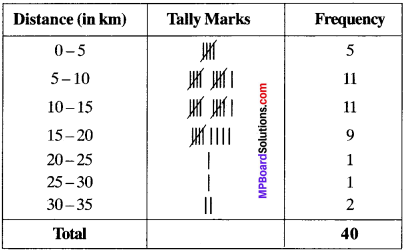We can observe that residence of most of the engineers are at a distance of 5 – 20 km from their place of work.

Question 3.
The relative humidity (in %) of a certain city for a month of 30 days was as follows: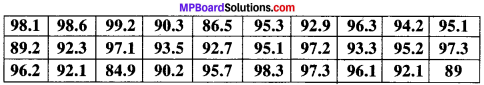1. Construct a grouped frequency distribution table with classes 84 – 86, 86 – 88, etc.
2. Which month or season do you think this data is about?
3. What is the range of this data?

Solution:
1. Frequency distribution table.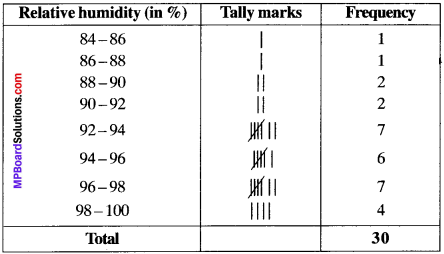2. Rainy season as the relative humidity is high.
3. Range = 99.2 – 84.9 .
= 14.3.

Question 4.
The heights of 50 students, measured to the nearest centimeters, have been found to be as follows: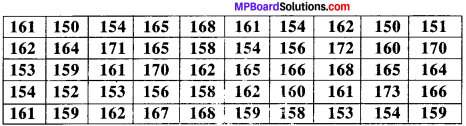1. Represent the data given above by a grouped frequency distribution table, taking the class intervals as 160 -165,165 -170, etc.
2. What can you conclude about their heights from the table?

Solution:
1. Frequency distribution table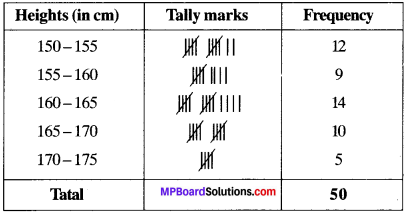2. We observe that most of the students have their heights between 160 – 165 cm.Question 5.
A study was conducted to find out the concentration of sulphur dioxide in the air in parts per million (ppm) of a certain city. The data obtained for 30 days is as follows: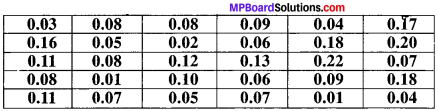1. Make a grouped frequency distribution table for this data with class intervals as 0.00 – 0.04, 0.04 – 0.08, and so on.
2. For how many days, was the concentration of sulphur dioxide more than 0.11 parts per million?

Solution:
1.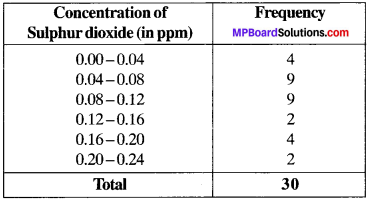2. The concentration of sulphur dioxide was more than 0.11 ppm for 8 days.

Question 6.
Three coins were tossed 30 times simultaneously. Each time the number of heads occurring was noted down as follows: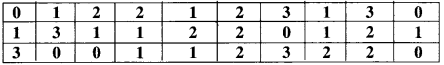Prepare a frequency distribution table for the data given above.
Solution: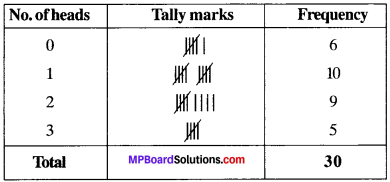Question 7.
The value of n upto 50 decimal places is given below:
3.14159265358979323846264338327950288419716939937510

1. Make a frequency distribution of the digits from 0 to 9 after the decimal point.
2. What are the most and the least frequently occurring digits?

Solution:
1.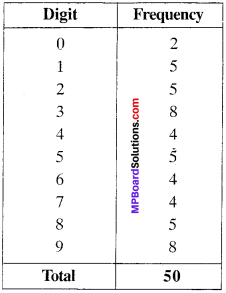2. The most frequently occurring digits are 3 and 9. The least occurring is 0.

Question 8.
Thirty children were asked about the number of hours they watched TV programmes in the previous week. The results were found as follows: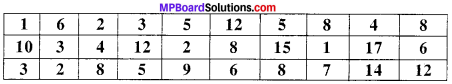1. Make a grouped frequency distribution table for this data, taking class width 5 and one of the class intervals as 5 – 10.
2. How many children watched television for 15 or more hours a week?

Solution:
1.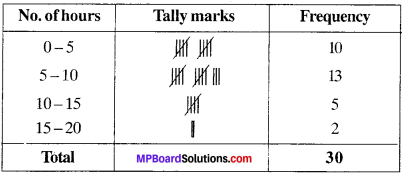2. 2 children.

Question 9.
A company manufactures car batteries of a particular type.ffhe lives (in years) of 40 such batteries were recorded as follows: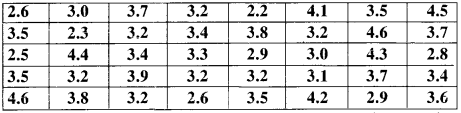Construct a grouped frequency distribution table for this data, using class intervals of size 0.5 starting from the interval 2 – 2.5.
Solution: